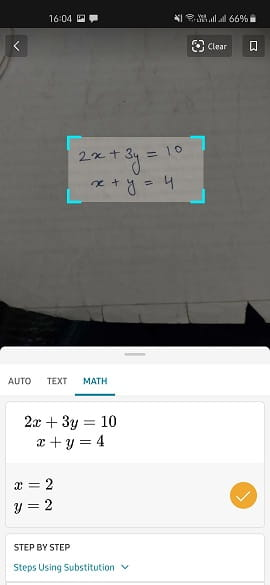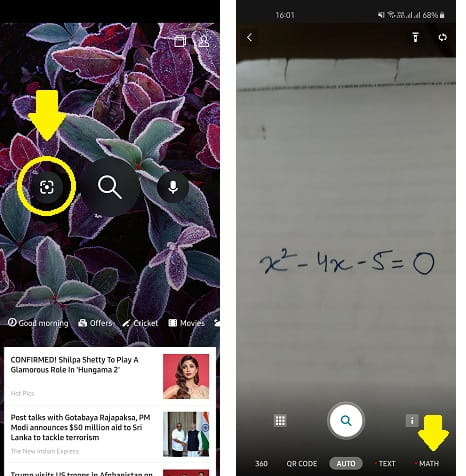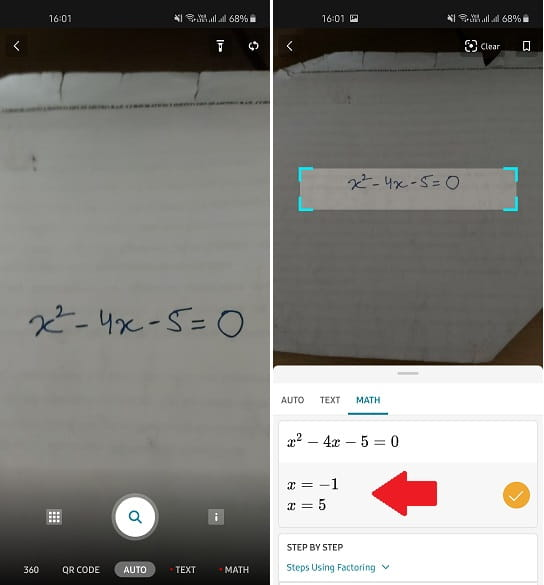Editor Ratings:
User Ratings:
[Total: 2 Average: 3.5]

This post aims to explain how to solve math equations by taking picture with Microsoft Math. Math is the new feature of the Bing app that you can use to solve mathematical equations with ease. With this, you can solve quadratic, linear, and general analytical equations. You only have to use the Bing’s image search feature and there is “Math” option that you can use to do it. It can solve the simple as well as complex equations in a matter of seconds. This is a very nice feature for you if you generally use mathematics in your daily life.

You can solve a math equation manually but it will take time if you do that with pen and paper. Or, in case, you don’t have a scientific calculator available then you can now take help of Bing app. You just have snap the math equation from anywhere and then you can simply let it solve for you. This is very simple and you don’t have to sign in or do something like that. Just scan the equation and get t solved correctly in a few seconds.## Ho to Solve Math Equations by Taking Pictures with Microsoft Math?

If you already using Bing app on your Android or iOS device then you just open the search. Or, you can opt to download the app from Play Store from this link. The Bing app is very powerful for searching content over internet. It even has AR search that you will like.

Now, just open the visual search in Bing and then tap on Math option from Bottom.Hold the camera steady and select the equation that you want to solve. Make sure to select the equation in the frame. The fame is re-sizable. After you click the photo, it will process the equation that you have captured and then you can simply see the results. It can precisely calculates the value of the variables in linear equations. See the screenshot below.In this way, you can make use of Microsoft Math to solve Mathematical equations with ease. You only have to get the app then you can simply start solving any kind of equation by just taking a picture of it. So, if you like mathematics then you can try this new app and then you can simply solve mathematical equations.

### Final thoughts

If you want to try a new way to solve math equations then you can try the new Bing app. Just use the Bing search in the usual way and then you can simply done. I liked the way it works and you will too if you use it once. The process of solving math is very easy, you just install it and then give it try. I tried it on linear and quadratic equations and it was able to solve them both precisely.

 Editor Ratings: User Ratings:[Total: 2 Average: 3.5] Free/Paid: Free